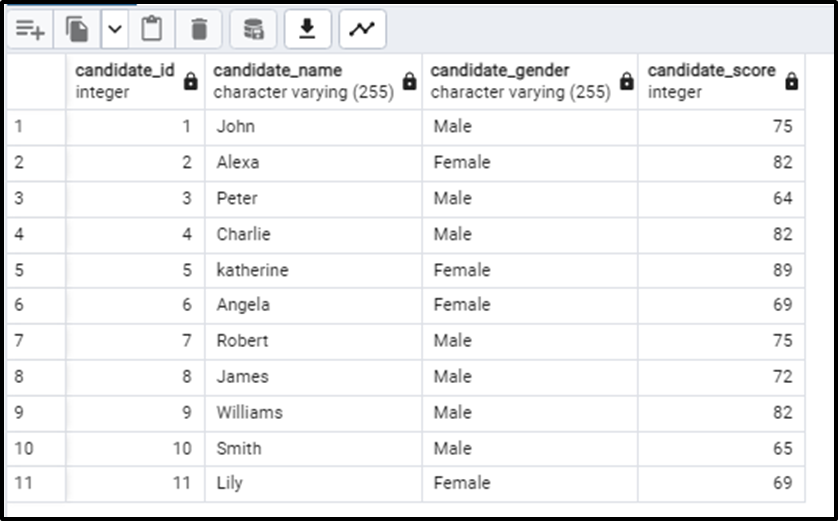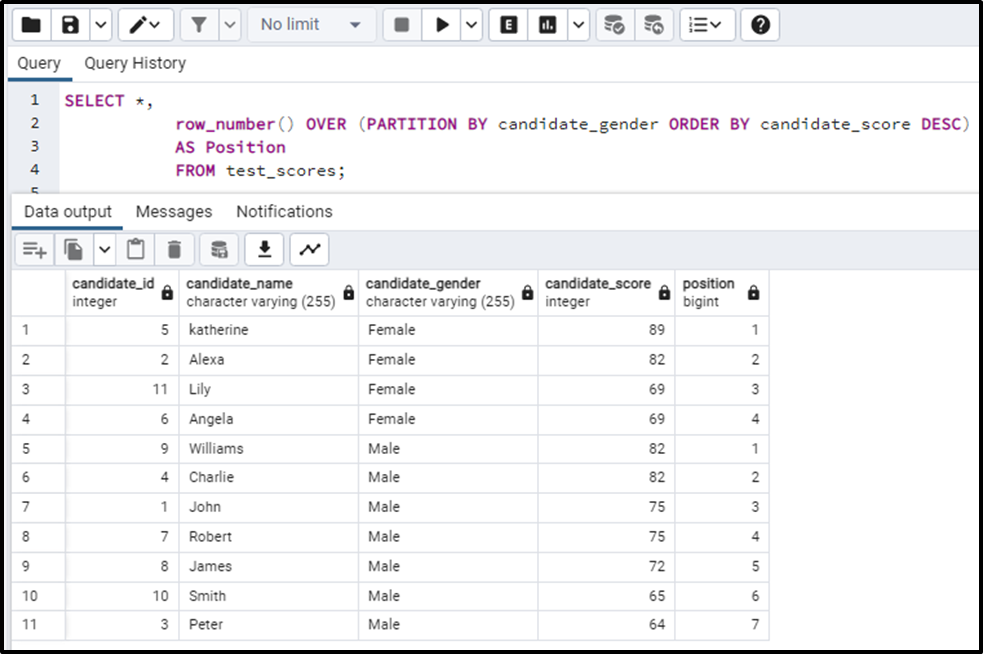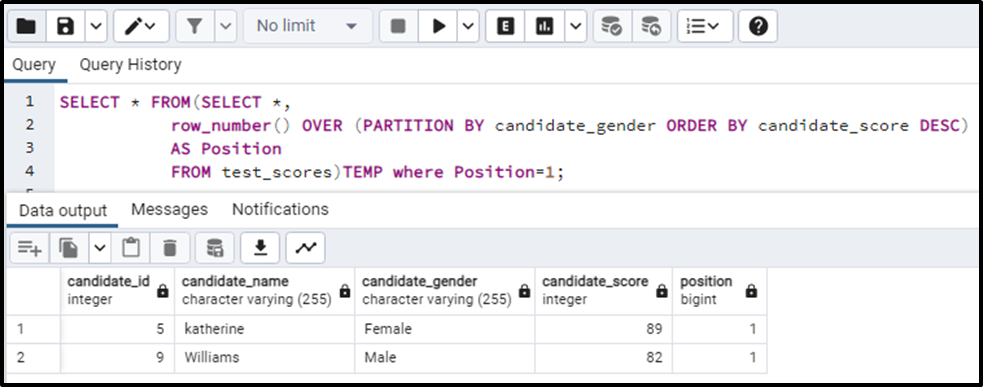##### How to Get the First Row Per Group in PostgreSQL

Getting the first row for each group can be of great use. This data can be used for many important purposes, especially for data analysis. For example, if we have a database table storing all the test scores for the candidates who appeared in the entrance test, by getting the first row for each group based on gender we can get the top scorer in both groups i.e. male and female.

This write-up will demonstrate the complete process of getting the first row per group in Postgres.

## How to Get the First Row Per Group in PostgreSQL

To get the first row per group, we will have to divide the data into groups or partitions. That partition can be based on anything we want our categories to be.

Let’s find out how to get the frost row for every group using suitable examples.

Example: Getting First Row Per Group

Let’s consider a database table, named “test_scores”, containing the data of the candidates who gave an entrance test for a university along with the scores they obtained on that test:Now if we want to know who got the first position in the category of male and female we need to get the first rows of both the groups. Right? Some things are generally clear:

- We will have to assign row numbers to the rows.

- We will have to make partitions.

And if we see it critically, the flow will be, we will have to separate the groups based on gender(partitioning) and assign row numbers to both groups separately, in order to get the first rows from both categories.

So to get the first row per group, we will have to write the following queries:

```SELECT *,
row_number() OVER (PARTITION BY candidate_gender ORDER BY candidate_score DESC)
AS Position
FROM test_scores;```

In the above query we have used:

- SELECT query to select/fetch data from the table “test_score”.

- row_number() function, which assigns row numbers to every row of the table.

- PARTITION BY divides the table data into groups. The PARTITION BY clause is followed by a column name on the base of which we want to partition the data.

- ORDER BY arranges the column, specify the name after it, in an order specified i.e. DESC means in descending order.

- The query till now, orders the data in descending order, partitions it based on gender i.e. Male and female, and then gives a row number to each row in both partitions, and this row number is stored in another column named as “Position”.

Output

We can see that the above table is now partitioned into 2 gender categories, and the row number has been assigned to both of the partitions separately:Since we only need the first row from both categories, we will have to modify the above code slightly like this:

```SELECT * FROM(SELECT *,
row_number() OVER (PARTITION BY candidate_gender ORDER BY candidate_score DESC)
AS Position
FROM test_scores)TEMP where Position = 1;```

Here we have basically specified that we need the data of row number/position 1. So the above query will give the first row per group:Note
: To get the last row per group, the above query will be the same. The only difference will be the ordering of the data i.e. we will order the data in ascending order using ASC instead of DESC. By doing so we will get the last person on the top.

### Conclusion

Getting specific data from the database tables is always of great use. This data can be used for analysis purposes. We can get the first row per group by arranging them in our preferred order, dividing them into partitions, giving each row a row number, and then retrieving the top row.